﻿ ElectricCharge <!--Induction_and_Inductance -->

## General Physics (calculus based) Class Notes

Dr. Rakesh Kapoor, M.Sc., Ph.D.

Former Faculty-University of Alabama at Birmingham, Birmingham, AL 35294
﻿

Electric Charge

Objectives

In this chapter we will introduce a new property of matter known as  “electric charge”

Learn about types of electric charge.

Explore the charge of atomic constituents.

Learn about Charge quantization & Conservation of Charge.

Learn about Electrostatic force among two charges (Coulomb’s law)

Electric Force and principle of superposition.

Why it happens?

When a plastic comb is rubbed with your hairs, it acquires the property of attracting light objects such as paper pieces.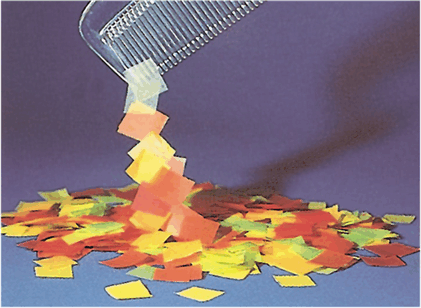If amber is rubbed on a cloth, it acquires the property of attracting light objects such as feathers, paper pieces.

When we rub a glass rod with silk cloth, it also acquires the property of attracting light objects such as feathers, paper.

Electric Charge:

This phenomenon was attributed to a new property of matter called “electric charge.”  (Electron is the Greek name for amber.)

There are two distinct types of electric charges:  positive (color code: red) and negative (color code: black).

The names “positive” and “negative” were given arbitrarily by Benjamin Franklin

How we came to the conclusion about two types of charge?

Charges with same electrical sign repel each other, and charges with opposite signs attract each other.

We can also say that charges are exerting force on each other. We call this force electrostatic force.

How can we check the type of charge on an object?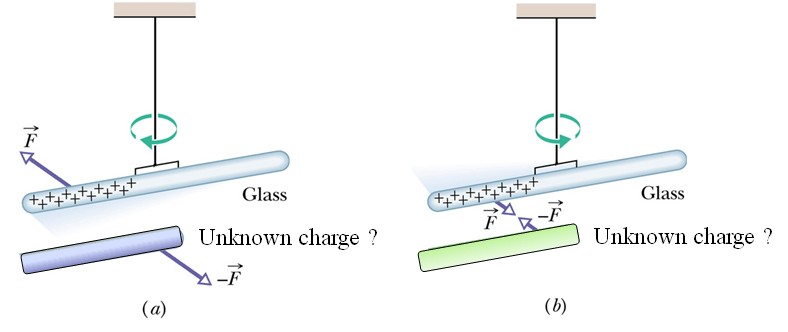Suppose we charge an object by rubbing it with another object or by any mean.

Now we want to know what type of charge (positive or negative) is there on this object.

First we can have a hanging Glass rods rubbed with silk cloth (We know the charge on glass rod is positive)

Now we bring the other charged object with unknown charge closer to this glass rod.

If unknown rod repels the hanging glass rod, we conclude that the unknown charge is positive

If unknown rod attracts the hanging glass rod, we conclude that the unknown charge is negative.

What is this charge?

At the beginning of 20th century Ernest Rutherford revealed that matter is made up of Atoms.

Atoms consist of electrons and the nucleus.

The nucleus itself consists of two types of particles: protons and neutrons.

The electrons are negatively charged.

The protons are positively charged.

The neutrons are neutral (zero charge).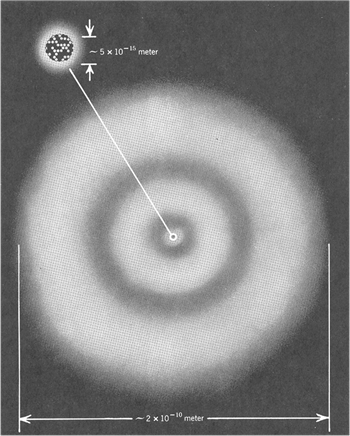Thus electric charge is a fundamental property of the elementary particles (electrons, protons, neutrons) out of which atoms are made.

Properties of Atoms:

Atoms are electrically neutral.

Typical Atoms have sizes.

Typical Nuclei have sizes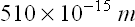.

In all the atoms, the number of electrons is equal to the number of protons.

This number is known as the “ atomic number ” (symbol: Z).

The chemical properties of atoms are determined exclusively by Z.

The sum of the number of protons and the number of neutrons is known as the “ mass number ” (symbol: A).

For  Uranium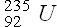,  number of proton/electron Z = 92  and number of protons + neutrons A = 235

Charge is Quantized

The net charge of an object containing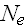numbers of electrons,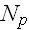numbers of protons and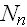numbers of neutron will be given as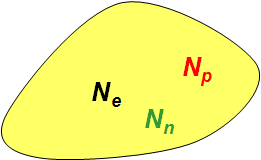Total number of charges,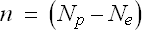is an integer.

We can say that charge on an object is always a multiple of elementary charge e. The value of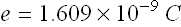.

In other words we can say that charge on any object is discrete or quantized. (It can not have a continuous value)

Quiz-1

Complete the following statement: When a glass rod is rubbed with silk cloth, the rod becomes positively charged as

(a) negative charges are transferred from the rod to the silk.

(b)  negative charges are transferred from the silk to the rod.

(c) positive charges are created on the surface of the rod.

(d)  positive charges are transferred from the silk to the rod.

(e)  positive charges are transferred from the rod to the silk.

Charge Conservation

Take a Glass rod and a silk cloth.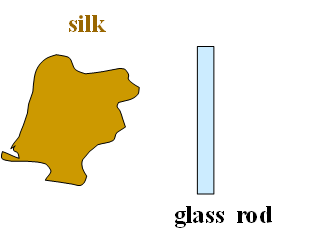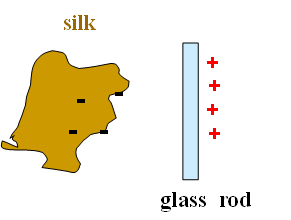Before charging the glass rod and silk cloth have equal number of protons and electrons.

After charging electrons move from glass rod to the silk cloth.

Silk cloth should have negative charge.

Net rod + cloth charge is still zero.

Rubbing does not produce charge, it only transfers charge from one object to other.

In any process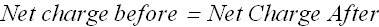Conductors & Insulators

Conductors are materials through which charge can move rather freely; examples include metals (such as copper in common lamp wire), the human body, and tap water.

Nonconductors are materials through which charge cannot move freely; examples include rubber , plastic, glass, and chemically pure water.

Conductors:

When atoms of a conductor like copper come together to form the solid, some of their outermost (and so most loosely held) electrons become free to wander about within the solid, leaving behind positively charged atoms (positive ions).

We call the mobile electrons conduction electrons.

In conducting solids (metals) electrons are mobile but in conducting solutions both positive and negative ions are mobile.

Checkpoint 1(Conservation of Charge):

Initially, sphere A has a charge of -50e and sphere B has a charge of +20e. The spheres are made of conducting material and are identical in size.
If the spheres then touch, what is the resulting charge on sphere A?.

Solution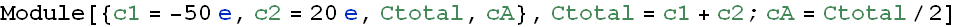How a conductor behaves in presence of a charged rod?

Consider a conducting rod (copper rod) hanging with an insulating thread. Copper rod is neutral as it contains equal number of electrons and protons.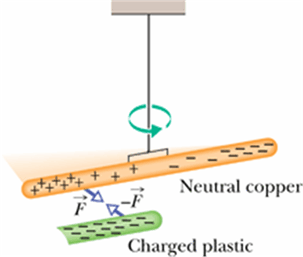Let us bring a negatively charged plastic rod close to it. (plastic rod rubbed with fur)

Since plastic rod is negatively charged, the free electrons in copper will get repelled by it and try to move away from the plastic rod side.

Now the side closer to the plastic rod has more positive charges.

Will the copper rod get attracted or repelled by the plastic rod?

It will get attracted. (see even a neutral conducting rod will get attracted to a charged plastic rod)
Induced charge is always opposite charge.

Although the copper rod is still neutral, it is said to have an induced charge, which means that some of its positive and negative charges have been separated due to the presence of a nearby charge.

Checkpoint 2 (Conductors and Insulators):

The figure shows five pairs of plates: A, B, and D are charged plastic plates and C is an electrically neutral copper plate. The electrostatic forces between the pairs of plates are shown for three of the pairs.
For the remaining two pairs, do the plates repel or attract each other.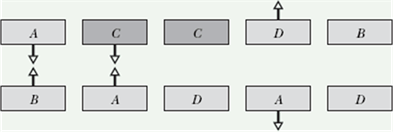Solution:

How to charge a conductor?

Consider a conducting rod (copper rod) hanging with an insulating thread. Copper rod is neutral as it contains equal number of electrons and protons.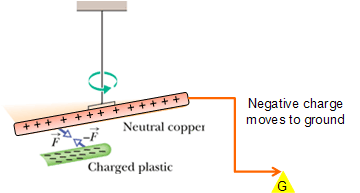In the presence of plastic rod if we connect the rod to ground with a conducting wire.

Only electron can move, therefore the electrons will move away to ground through the wire.

Now disconnect the wire while plastic rod is present.

The copper wire will get positively charged.

Thus, an object becomes positively charged only through the removal of negative charges.

What will  happen if we first remove the charged plastic rod and the disconnect the wire?

Quiz-2

An initially electrically neutral conducting sphere is placed on an insulating stand.  A negatively-charged glass rod is brought near, but does not touch the sphere.  Without moving the rod, a wire is then attached to the sphere that connects it to earth ground.  The rod and wire are then removed simultaneously.  What is the final charge on the sphere?

(a) negative

(b)  positive

(c)  neutral

(d)  It has a fifty percent chance of having a positive charge and a fifty percent chance of having a negative charge.

Quiz-3

Three identical conducting spheres on individual insulating stands are initially electrically neutral.  The three spheres are arranged so that they are in a line and touching as shown.  A negatively-charged conducting rod is brought into contact with sphere A.  Subsequently, someone takes sphere C away.  Then, someone takes sphere B away.  Finally, the rod is taken away.  What is the sign of the final charge, if any, of the three spheres?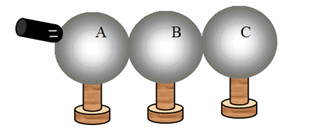A    B    C

(a)           +          +           -

(b)            +          -           +

(c)            +          0           -

(d)           -            +          0

(e)         -             -           -

Quiz-4

Three insulating balls are hung from a wooden rod using thread.  The three balls are then individually charged via induction.  Subsequently, balls A and B are observed to attract each other, while ball C is repelled by ball B.  Which one of the following statements concerning this situation is correct?

(a) A and B are charged with charges of opposite signs; and C is charged with charge that has the
same sign as B.

(b) A and B are charged with charges of the same  sign; and C is electrically neutral.

(c)  A is electrically neutral; and C is charged with charge that has the same sign as B.

(d) B is electrically neutral; and C is charged with charge that has the same sign as A.

(e) Choices a and c are both possible configurations.

Direction and magnitude of Electrostatic force.

Watch the direction of electrostatic force (red arrows) between two charges held at a distance from each other.

Now move position of charges (click hold and drag the sphere) and see the direction and magnitude (arrow length) of force.

Now change the (by respective slider) charge on any of the sphere watch the direction and magnitude (arrow length) of force.

What we observed?

Coulomb’s Law

These experimental observations gave us following relation between electrostatic force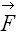, charges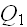and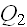and distance r between the charges.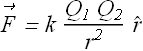Where k is a constant with following value.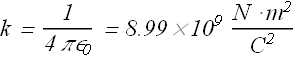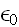is called the permittivity constant, its value is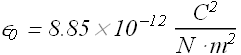Unit of Charge:

In SI system the unit of charge is Coulomb

Symbol for the unit is “C”

It is derived from the unit of current (Ampere).

Current is the flow of charge through a conductor in one secondTherefore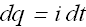Unit of current in SI system is Ampere and symbol is “A”.

Therefore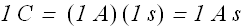Electrostatic Force and principle of superposition

Among the following two charges the electrostatic force on chargedue to chargeis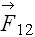.

Let us add more charges in the figure (at any location press Alt-Click).

Observe the net force arrow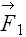(thick brown) on charge.

What we observe?

The net electrostatic forceon chargeis vector sum of electrostatic forces due to all other charges.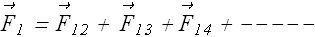If there are n+1 charges, forceon charge 1 due to all other n charges is given as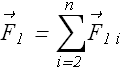We conclude that electrostatic force on a charge follow principle of superposition. Means force on any one charge is vector sum of individual forces on that charges due to all other charges present.

Checkpoint 3 (Coulomb’s Law):

The figure shows two protons (symbol p) and one electron (symbol e) on an axis.
What is the direction of (a) the electrostatic force on the central proton due to the electron, (b) the electrostatic force on the central proton due to the other proton, and (c) the net electrostatic force on the central proton?Solution:

Electrostatic Force and Shell theorem:

A shell of uniform charge attracts or repels a charged particle that is outside the shell as if all the shell's charge were concentrated at its center.

Out side uniformly charged shell the force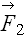onis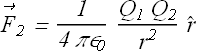If a charged particle is located inside the uniformly charged shell, there is no net electrostatic force on the particle from the shell.

Inside a uniformly charged shell, forceonis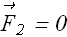Checkpoint 4 (Coulomb’s Law):

The figure here shows three arrangements of an electron e and two protons p.
(a) Rank the arrangements according to the magnitude of the net electrostatic force on the electron due to the protons, largest first.
(b) In situation c, is the angle between the net force on the electron and the line labeled d less than or more than 45°?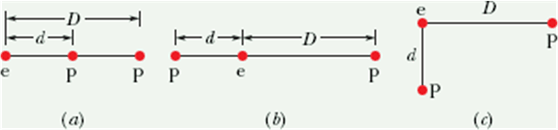Motion of charged cloud in presence of another charged cloud.

These are two spherical charge clouds. One is fixed and other can be released.
Change the distance between the charges (click any charge hold and drag) and watch their motion when released (click play).

Change the charge onorand watch the motion after releasing the charges.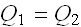, but chargeis thrown towardswith initial velocity.